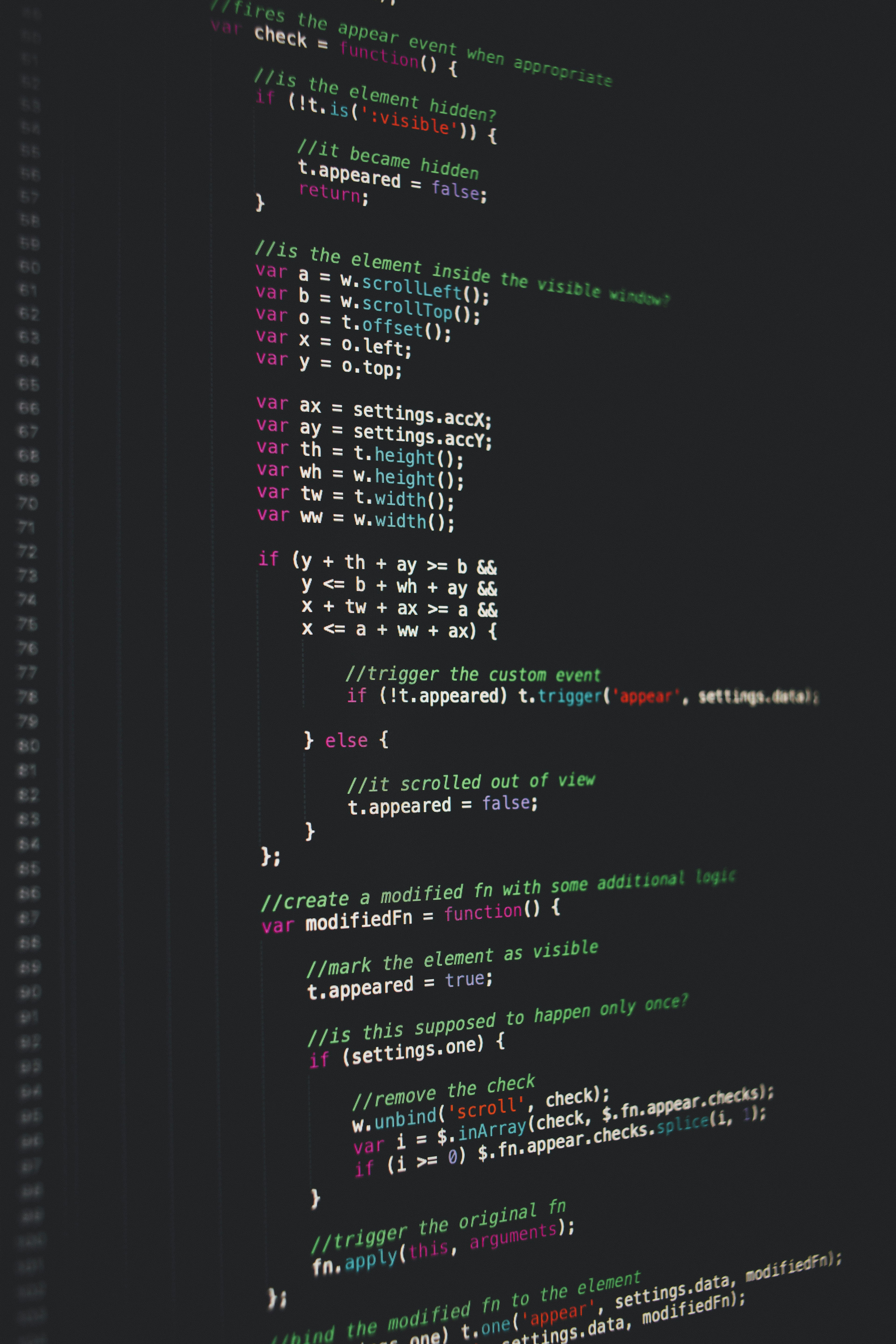Hackerrank Lower Bound-STL Solution

# Hackerrank Lower Bound-STL Solution.MathJax_SVG_LineBox {display: table!important} .MathJax_SVG_LineBox span {display: table-cell!important; width: 10000em!important; min-width: 0; max-width: none; padding: 0; border: 0; margin: 0}

You are given  integers in sorted order. Also, you are given  queries. In each query, you will be given an integer and you have to tell whether that integer is present in the array. If so, you have to tell at which index it is present and if it is not present, you have to tell the index at which the smallest integer that is just greater than the given number is present.

Lower bound is a function that can be used with a sorted vector. Learn how to use lower bound to solve this problem by Clicking Here.

Input Format.MathJax_SVG_LineBox {display: table!important} .MathJax_SVG_LineBox span {display: table-cell!important; width: 10000em!important; min-width: 0; max-width: none; padding: 0; border: 0; margin: 0}

The first line of the input contains the number of integers . The next line contains  integers in sorted order. The next line contains , the number of queries. Then  lines follow each containing a single integer .

Note that if the same number is present multiple times, you have to print the first index at which it occurs. Also, the input is such that you always have an answer for each query.

Constraints.MathJax_SVG_LineBox {display: table!important} .MathJax_SVG_LineBox span {display: table-cell!important; width: 10000em!important; min-width: 0; max-width: none; padding: 0; border: 0; margin: 0}

,where  is  element in the array.

Output Format.MathJax_SVG_LineBox {display: table!important} .MathJax_SVG_LineBox span {display: table-cell!important; width: 10000em!important; min-width: 0; max-width: none; padding: 0; border: 0; margin: 0}

For each query you have to print "Yes" (without the quotes) if the number is present and at which index(1-based) it is present separated by a space.

If the number is not present you have to print "No" (without the quotes) followed by the index of the next smallest number just greater than that number.

You have to output each query in a new line.

Sample Input

 8
1 1 2 2 6 9 9 15
4
1
4
9
15


Sample Output

 Yes 1
No 5
Yes 6
Yes 8


### Solution in cpp

Approach 1.

#include <cmath>
#include <cstdio>
#include <vector>
#include <iostream>
#include <algorithm>
using namespace std;
int main()
{
vector<long> v;
long n,q,y,i,inp,pos;
cin>>n;
for(i=0;i<n;i++)
{
cin>>inp;
v.push_back(inp);
}
sort(v.begin(),v.end());
cin>>q;
for(i=0;i<q;i++)
{
cin>>y;
pos=lower_bound(v.begin(),v.end(),y)-v.begin();
cout<<(v.at(pos)==y?"Yes":"No")<<" "<<pos+1<<endl;
}
return 0;
}


Approach 2.

#include <cmath>
#include <cstdio>
#include <vector>
#include <iostream>
#include <algorithm>
using namespace std;

int main() {
/* Enter your code here. Read input from STDIN. Print output to STDOUT */
int m, num;
cin >> m;
vector<int> v;
for (int i=0; i<m; i++){
cin >> num;
v.push_back(num);
}
int n, val;
cin >> n;
for (int i=0; i<n; i++){
cin >> val;
vector<int>::iterator low = lower_bound(v.begin(), v.end(), val);
if (v[low - v.begin()] == val)
cout << "Yes " << (low - v.begin()+1) << endl;
else
cout << "No " << (low - v.begin()+1) << endl;
}
return 0;
}


Approach 3.

#include <cmath>
#include <cstdio>
#include <vector>
#include <iostream>
#include <algorithm>
using namespace std;

int main() {
/* Enter your code here. Read input from STDIN. Print output to STDOUT */
int N, n;
cin >> N;
vector<int> v;
v.reserve(N);

for (int i = 0; i < N; ++i)
{
cin >> n;
v.push_back(n);
}

int Q, q;
cin >> Q;

for (int i = 0; i < Q; ++i)
{
cin >> q;
auto low = lower_bound(v.begin(), v.end(), q);
int pos = low - v.begin();
cout << (v[pos] == q ? "Yes " : "No ") << (pos + 1) << endl;
//Why above works but below does not?
//cout << (v[pos] == q ? "Yes " : "No ") << ++pos << endl;
}

return 0;
}